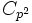# Fully invariant not implies verbal in finite abelian group

(diff) ← Older revision | Latest revision (diff) | Newer revision → (diff)
This article gives the statement and possibly, proof, of a non-implication relation between two subgroup properties, when the big group is a finite abelian group. That is, it states that in a finite abelian group, every subgroup satisfying the first subgroup property (i.e., fully invariant subgroup) need not satisfy the second subgroup property (i.e., verbal subgroup)
View all subgroup property non-implications | View all subgroup property implications

## Statement

A fully characteristic subgroup of a finite abelian group need not be a verbal subgroup.

## Proof

### Example of a group of prime-cube order

Let$C_p$ and$C_{p^2}$ denote the cyclic groups of order$p$ and$p^2$ respectively. Let$G = C_p \times C_{p^2}$. Consider the omega-1 subgroup:$\Omega_1(G) := \{ x \in G \mid px = 0 \}$.

In other words, it is the subgroup of$G$ comprising the elements of order dividing$p$. Then,$\Omega_1(G)$ is fully characteristic: any endomorphism of$G$ preserves the condition. On the other hand,$\Omega_1(G)$ is not verbal: the only possible verbal subgroups of$G$ are the agemo subgroups: the whole group$G$, the set of multiples of$p$, and the trivial subgroup. None of these equals$\Omega_1(G)$.